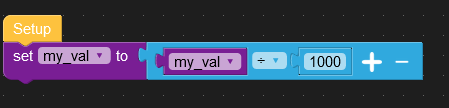# Int to Float conversion

• Hello, how can I convert an int value (10000 mV) from a variable to a float value in a Label block (10,000 V) ?
Thank you all for the help

• What language?

In Python you can simply:

my_val = 10000
my_val = my_val/1000

• Since you posted under UIFlow, then that is what you use I suppose:which results in the following Python code:

my_val = my_val / 1000

• thank you Cognitive5525, I have already tried but it does not work.

Here is the partial code:

``````  A3 = (str((hex(A2)[2:] + hex(A1)[2:])))
A4 = (str(int(A3, 16))) // here all ok and the Label shows 10000 mV
A5 = A4 / 1000
label0.setText(str(A5)) //the label shows nothing``````

• I'm not sure what A3 becomes since I can't see what A1 and A2 are, but:

A4 = (str(int(A3, 16)))

makes A4 a string which you can't do arithmetic on.

Provided that A3 can be converted to an integer, I would:

A4 = int(A3, 16)
A5 = A3 /1000
label0.setText(str(A5))

• A4 = int(A3, 16)
A5 = A3 /1000
label0.setText(str(A5))

Thank you!

solved with this code:

A4 = int(A3, 16)
A5 = A4 /1000
label0.setText(str(A5))

• Use the math function block to convert in to float then use a text block to convert to string?